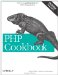# Recipe 4.23. Finding the Union, Intersection, or Difference of Two Arrays

### Recipe 4.23. Finding the Union, Intersection, or Difference of Two Arrays

#### 4.23.1. Problem

You have a pair of arrays, and you want to find their union (all the elements), intersection (elements in both, not just one), or difference (in one but not both).

#### 4.23.2. Solution

To compute the union:

`\$union = array_unique(array_merge(\$a, \$b));`

To compute the intersection:

`\$intersection = array_intersect(\$a, \$b);`

To find the simple difference:

`\$difference = array_diff(\$a, \$b);`

And for the symmetric difference:

`\$difference = array_merge(array_diff(\$a, \$b), array_diff(\$b, \$a));`

#### 4.23.3. Discussion

Many necessary components for these calculations are built into PHP; it's just a matter of combining them in the proper sequence.

To find the union, you merge the two arrays to create one giant array with all of the values. But array_merge( ) allows duplicate values when merging two numeric arrays, so you call array_unique( ) to filter them out. This can leave gaps between entries because array_unique( ) doesn't compact the array. It isn't a problem, however, as foreach and each( ) handle sparsely filled arrays without a hitch.

The function to calculate the intersection is simply named array_intersection( ) and requires no additional work on your part.

The array_diff( ) function returns an array containing all the unique elements in \$old that aren't in \$new. This is known as the simple difference:

`\$old = array('To', 'be', 'or', 'not', 'to', 'be'); \$new = array('To', 'be', 'or', 'whatever'); \$difference = array_diff(\$old, \$new); \$old = array('To', 'be', 'or', 'not', 'to', 'be'); \$new = array('To', 'be', 'or', 'whatever'); \$difference = array_diff(\$old, \$new); Array (      => not      => to )`

The resulting array, \$difference contains 'not' and 'to' because array_diff( ) is case-sensitive. It doesn't contain 'whatever' because it doesn't appear in \$old.

To get a reverse difference, or in other words, to find the unique elements in \$new that are lacking in \$old, flip the arguments:

`\$old = array('To', 'be', 'or', 'not', 'to', 'be'); \$new = array('To', 'be', 'or', 'whatever'); \$reverse_diff = array_diff(\$new, \$old); \$old = array('To', 'be', 'or', 'not', 'to', 'be'); \$new = array('To', 'be', 'or', 'whatever'); \$reverse_diff = array_diff(\$new, \$old); Array (      => whatever )`

The \$reverse_diff array contains only 'whatever'.

If you want to apply a function or other filter to array_diff( ), roll your own diffing algorithm:

`// implement case-insensitive diffing; diff -i \$seen = array(); foreach (\$new as \$n) {     \$seen[strtolower(\$n)]++; } foreach (\$old as \$o) {     \$o = strtolower(\$o);     if (!\$seen[\$o]) { \$diff[\$o] = \$o; } }`

The first foreach builds an associative array lookup table. You then loop through \$old and, if you can't find an entry in your lookup, add the element to \$diff.

It can be a little faster to combine array_diff( ) with array_map( ):

`\$diff = array_diff(array_map('strtolower', \$old), array_map('strtolower', \$new));`

The symmetric difference is what's in \$a but not \$b, and what's in \$b but not \$a:

`\$difference = array_merge(array_diff(\$a, \$b), array_diff(\$b, \$a));`

Once stated, the algorithm is straightforward. You call array_diff( ) twice and find the two differences. Then you merge them together into one array. There's no need to call array_unique( ) since you've intentionally constructed these arrays to have nothing in common.

Documentation on array_unique( ) at http://www.php.net/array-unique, array_intersect( ) at http://www.php.net/array-intersect, array_diff( ) at http://www.php.net/array-diff, array_merge( ) at http://www.php.net/array-merge, and array_map( ) at http://www.php.net/array-map .PHP Cookbook: Solutions and Examples for PHP Programmers
ISBN: 0596101015
EAN: 2147483647
Year: 2006
Pages: 445

Similar book on Amazon